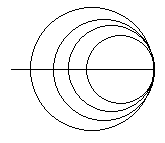# Electronics and Communication Engineering - Electromagnetic Field Theory

46.
A λ/8 section of transmission line is determined of load impedance of (2 - j 5) Ω. If characteristic impedance of line is 10 Ω. Then input impedance of line is
10 Ω
(6.9 + j 7.24) Ω
(1.75 + j 3.098) Ω
(2 - j 5) Ω
Explanation:
No answer description is available. Let's discuss.

47.
line of sight
ionospheric wave
ground wave
skywave
Explanation:
No answer description is available. Let's discuss.

48.
Many circles are drawn in a Smith chart used for transmission line calculations. The circle shown in the figure representsunit circles
constant resistance circles
constant reactance circles
constant reflection coefficient circles
Explanation:
No answer description is available. Let's discuss.

49.
A loop antenna is a commonly used for
direction finding
satellite communication
all of the above